# Analytics Calculations: Logic Functions

Moderator, Lightspeed Staff Posts: 81 moderator
edited June 2018
*Note, from this part of the Discussions, we will assume that you're comfortable with starting Calculations. Changing dimensions and measures, changing filters, and some of the basic starter calculations: addition, subtraction, division, multiplication, greater than, less than, equal to, single-criteria IF functions, and multiple criteria IF functions.

If you're in the early stages of your Analytics mastery, you may want to take a quick look at the related Discussions:

Multiplying by percentages in Analytics

Analytics provides lots of functions that perform different mathematical, logical, and table-based calculations. You can find a more in-depth catalogue of available functions on the Looker list of functions and operators.

To make it easier, we could categorize these in five different ways: Cell functions, Date functions, Logic functions, Math functions, and Table functions

In this article, we'll be looking at seven of the Logic based functions:
And, is_null, No, Not, Or, Row, Yes

# And

Used to return a result if two or more sets of criteria are met

In this example, I'm looking for instances where the Sales Date was "Monday" and where the Category of Sale was "Bikes"

We need at least two sets of criteria defined as IF functions. In this case, the positive and negative responses must be "yes" and "no"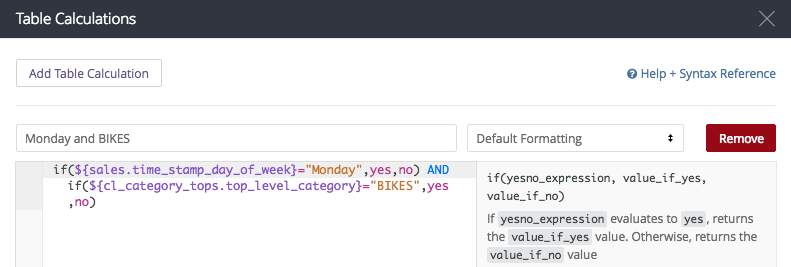My calculation here looks like:
if(\${sales.time_stamp_day_of_week}="Monday",yes,no) AND if(\${cl_category_tops.top_level_category}="BIKES",yes,no)

And if I have saved my calculation properly...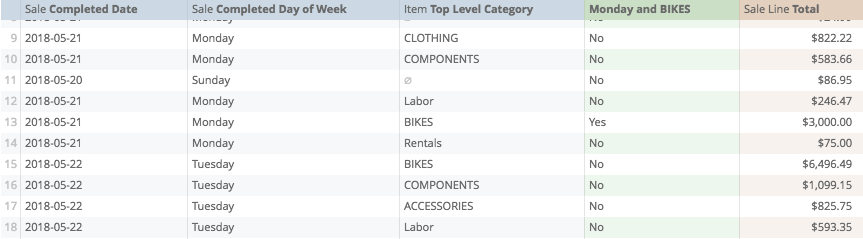# is_null

Returns whether a field is null. Requires one argument, the field it is searching:

For example, if I want to find days with sales of products with no category.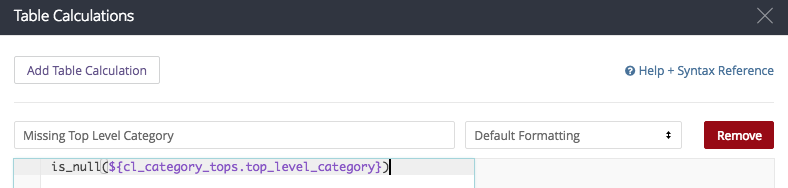my calculation looks like:
is_null(\${cl_category_tops.top_level_category})

and when I save...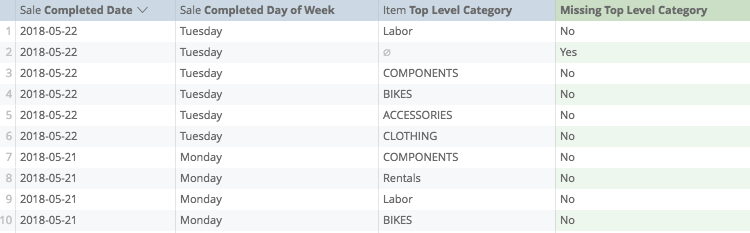# No

"No" is not a defined function, but is a response to logical calculations (such as "is_null"), which can be used in compound calculations. We'll look at this more in later discussions, but for now.

In this instance, I'm asking Analytics to find instances of Categories being null. If they are not, I'm asking Analytics to return a 1, if so, return a 0.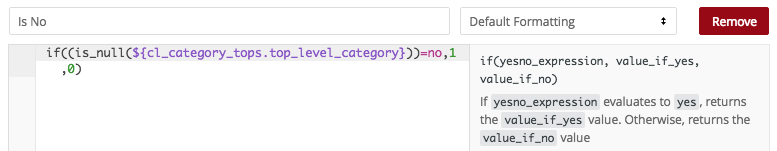My calculation looks like:
if((is_null(\${cl_category_tops.top_level_category}))=no,1,0)

and if I have saved my calculation properly...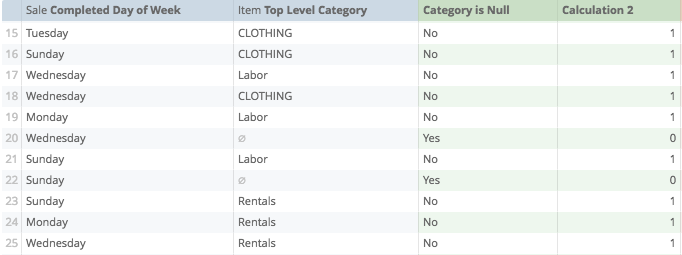# Not

"Not" returns the inverse results of what is asked for in "Yes" or "No" results.

To illustrate: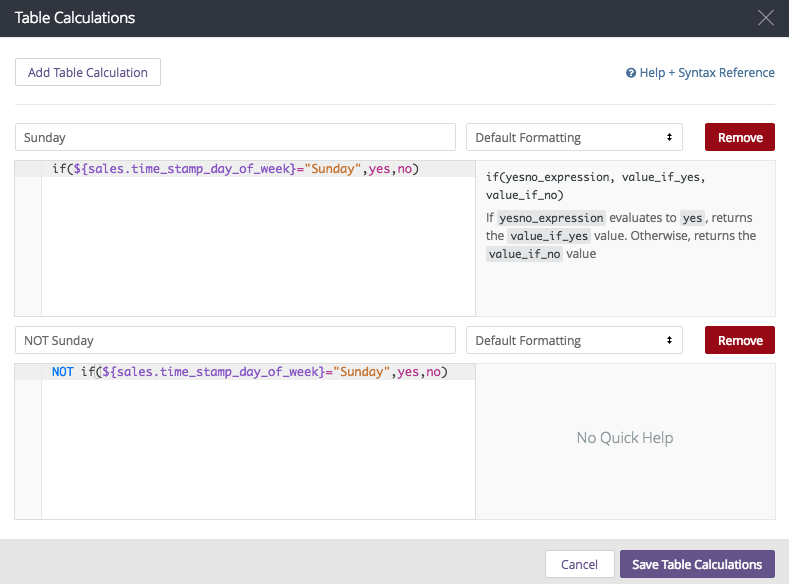My calculation looks like:
NOT if(\${sales.time_stamp_day_of_week}="Sunday",yes,no)

and if I've prepared my calculation properly...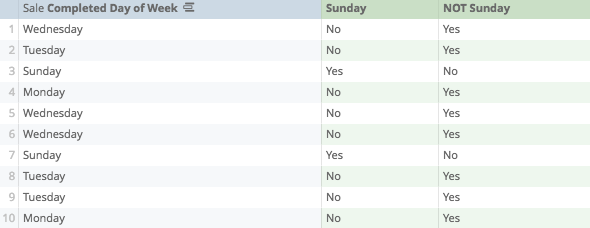# Or

Like "And", used to return a result if at least one of two or more arguments is fulfilled.

For example, in this calculation, we're looking for instances where the Day of the Sale was Monday, or the Category of the Sale was "Bikes"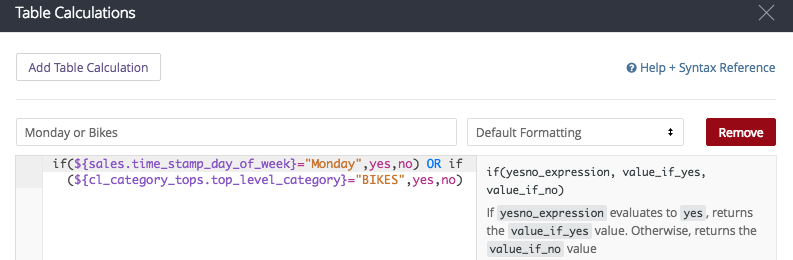so my calculation looks like:

if(\${sales.time_stamp_day_of_week}="Monday",yes,no) OR if(\${cl_category_tops.top_level_category}="BIKES",yes,no)

and if I've done my calculation properly, then when I save...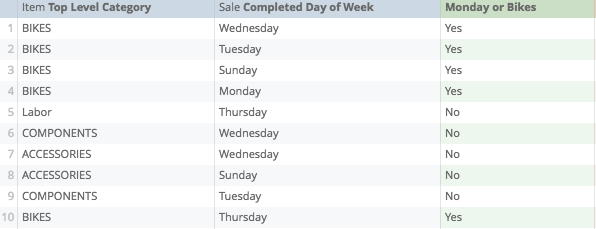# Row

Returns the number corresponding to the row on the table. No argument required.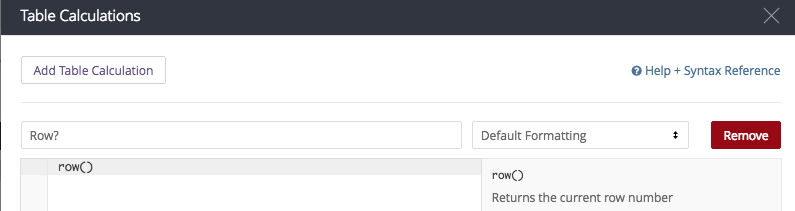My calculation looks like:

row()

and if I have prepared it properly, when I save...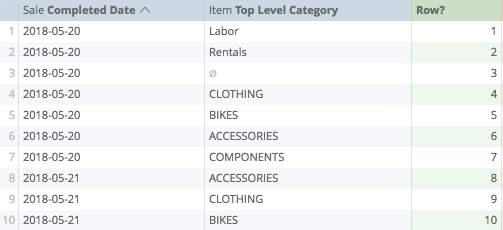# Yes

Like "No", is a response to logical calculations (such as "is_null"), which can be used in compound calculations.

In the converse example of "No", I'm looking for instances where the Product Category is null: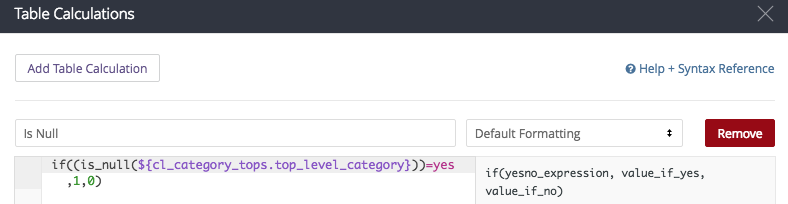So, my calculation looks like:

if((is_null(\${cl_category_tops.top_level_category}))=yes,1,0)

and if I have prepared it properly...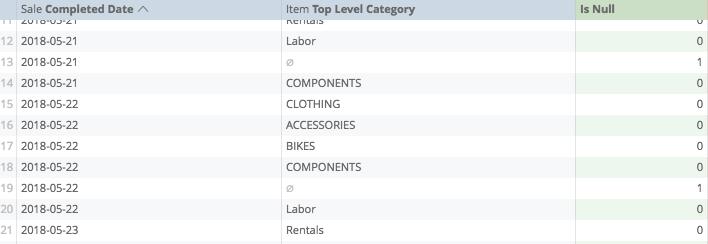Calculations Home
Post edited by josephmckeown on
Joseph McKeown
Lightspeed Analytics and reporting consultant
Lightspeed HQ